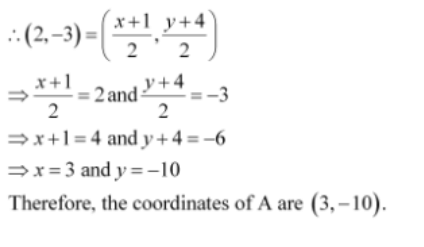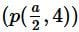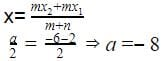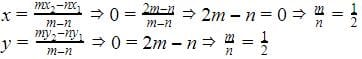# Test: Section Formula

## 15 Questions MCQ Test Online MCQ Tests for Class 10 | Test: Section Formula

Description
Attempt Test: Section Formula | 15 questions in 15 minutes | Mock test for Class 10 preparation | Free important questions MCQ to study Online MCQ Tests for Class 10 for Class 10 Exam | Download free PDF with solutions
QUESTION: 1

### The coordinates of a point A, where AB is diameter of a circle whose centre is (2,-3) and B is (1, 4), are:​

Solution:

Let the co-ordinates of point A is (x, y)

Now, AB is the diameter of circle. So, mid-point of AB is the center of the circle.

Given, center O(2,-3) and B is (1,4).

So, by using mid-point formula, we get:QUESTION: 2

### Ifis the mid-point of the line-segment joining the points A (-6, 5) and B (-2,3) then the value of a is

Solution:

Applying distance formulaQUESTION: 3

### The ratio in which the point P(-3,y) divides the line segment joining the points A(-5,-4) and B(-2,3) is​

Solution:
QUESTION: 4

The coordinates of the point which divide the line segment joining P (-2, 2) and Q (2, 8) into two equal parts are:​

Solution:
QUESTION: 5

The mid-point of the line segment joining P(-2,8) and Q(-6,-4) is

Solution:
QUESTION: 6

Determine the ratio in which the line 2x+y-4 = 0 divides the line segment joining the points A (2,-2) and B (3, 7)​

Solution:
QUESTION: 7

The mid point of the line segment joining A(2a,4) and B(-2,3b) is M (1,2a + 1). The values of a and b are​

Solution:
QUESTION: 8

The ratio in which the x-axis divides the segment joining A(3,6) and B(12,-3) is​

Solution:
QUESTION: 9

The ratio in which the line 2x+y-4 = 0 divides the line segment joining A(2,-2) and B(3,7) is​

Solution:
QUESTION: 10

If A (1,2) , B (4,y), c (x,6) and D (3,5) are the vertices of a parallelogram taken in order then the values of x and y are:​

Solution:
QUESTION: 11

The ratio in which the line segment joining A(3,4) and B(-2,1) is divided by the y-axis is

Solution:
QUESTION: 12

The line segment joining A(-2,9), and B(6,3) is a diameter of a circle with centre C. The co-ordinates of C are​

Solution:
QUESTION: 13

The coordinates of the point which divides the line segment joining points A(5,-2) and B(9,6) in the ratio 3:1 are​

Solution:
QUESTION: 14

The ratio in which (4,5) divides the line segment joining the points (2,3) and (7,8) is​

Solution:
QUESTION: 15

Origin divides the join of points (1,1) and (2,2) externally in the ratio​

Solution:

We have external section formula asUse Code STAYHOME200 and get INR 200 additional OFF Use Coupon Code